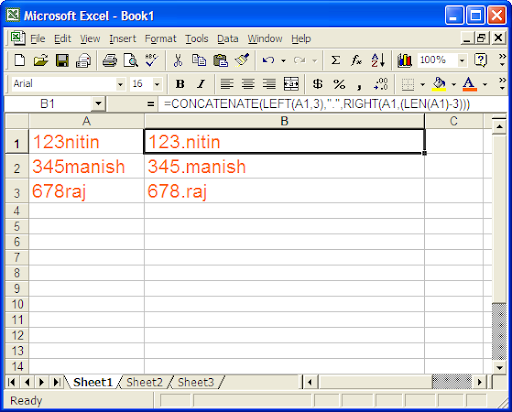## Jan 30, 2009

### Picture inside the laptop CD-ROM:

READ MORE - Picture inside the laptop CD-ROM:

## Jan 29, 2009

### Microsoft Excel Functions (Case Study Of Solving a Problem):

This morning I have came across a problem that is to be solved in Microsoft Excel using its functions, one of my friend bring to me. Here I am going to describe that how I have solved the problem.

What is the Problem?
I have a column in Excel that is column A. And data is feed in this column like given below.

123nitin
345manish
678raj

Now it requires that a dot (.) is to be placed in between the numeric and character as shown below.

123.nitin
345.manish
678.rajHow I solved the above problem?
I have solved the problem by using the functions as follows.
Left function, Right function, Concatenate functions.
So I have made a formula and put it in cell B1.

=Concatenate(LEFT(A1,3),".",RIGHT(A1,(LEN(A1)-3)))

Drag the handle and copy it to all required cells.

How the above formula is working?
Left(A1,3) will yield 123 for cell A1.
Right(A1,(LEN(A1)-3))) will yield nitin for cell A1.
Concatenate function will concatenate 123, . , nitin. So finally we get the String 123.nitin.
READ MORE - Microsoft Excel Functions (Case Study Of Solving a Problem):